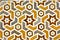# Breaking Down Compound Procedures in Lisp

## With a sprinkle of JavaScriptPhoto by Koushik Chowdavarapu on Unsplash
1. Means of combination: The way we combine those primitive objects, or the method “by which compound elements are built from simpler ones.”
2. Means of abstraction: I take this to mean a way of building on smaller elements of programming to build larger, more complex programs. In other words, using smaller building blocks to make bigger ones.
`(define (square x) (* x x))`
`(define (square  x)        (*         x     x)) To      square something, multiply   it by itself.`
`function square(x) { return x * x;}`
`(define (sum-of-squares x y) (+ (square x) (square y)))`
1. Tells it which parameters will go into it: `x y`
2. And then what will happen when he invokes the `sum-of-squares` procedure: `(+ (square x) (square y))`
`function sumOfSquares(x, y) { return square(x) + square(y);}`
`(define (f a)  (sum-of-squares (+ a 1) (* a 2)))`
1. Give it a parameter: `a`
2. Then run the `sum-of-squares` procedure on `a` as follows: `((+ a 1) (* a 2))`
`// Feed in the number 5:(sum-of-squares (+ 5 1) (* 5 2))// Before squaring:(sum-of-squares (6) (10))// After squaring:(sum-of-squares (36) (100))// After running the sum-of-squares procedure and "summing" them:136`
`function f(a) { return square(a + 1) + square(a * 2);}`

Coding, creativity, music, and books. Pianist & composer — @vontmer

## More from Joe Cardillo

Coding, creativity, music, and books. Pianist & composer — @vontmer